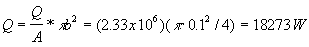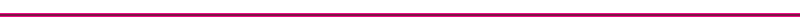Engine Heat Transfer Correlations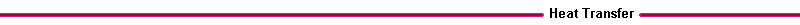For the overall average heat transfer from the gas to the cylinder coolant, convection type heat transfer equations are used.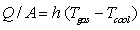Where:

Q = overall heat transfer (W/m2)
A = reference cylinder area (m2)
Tgas = effective gas temperature, typically 800 C
Tcool = coolant temperature, typically 80 C
h = heat transfer coefficient (W/m2 K)

The heat transfer coefficient depends on the engine geometry, such as the exposed cylinder area and bore, and the piston speed. Due to the complex gas flow in the cylinder, it varies with location in the cylinder and in time with changing piston position. The value of the heat transfer coefficient is found from a Nusselt number - Reynolds number type correlation.

Nusselt # = a (Reynolds #)m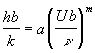Where:

b = cylinder characteristic length (m), usually chosen to be the cylinder bore.
k = gas thermal conductivity, (W/mK), typical value 0.06
n = gas kinematic viscosity, (m2/s), typical value 100 x 10-6
U = characteristic gas velocity (m/s)

The characteristic gas velocity U is typically the mean piston speed: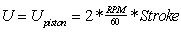However, some correlations use the average intake velocity: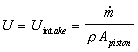and other correlations require a characteristic combustion velocity. The coefficient a and exponent m are found from engine experiments. There are three types of heat transfer coefficients used in the computation of engines heat transfer.

Table III. Types of Heat Transfer Coefficients
 Averaging Used for h(x,t) averaged over time & space overall steady state energy balance calculations h(x,t) instantaneous, space average heat transfer versus crank angle h(x,t) instantaneous, local local calculations of thermal stress

The peak values of the instantaneous and local coefficients can be many times higher than the averaged values.

A frequently used correlation for a space and time averaged heat transfer coefficient is that of C. F. Taylor ("The Internal Combustion Engine in Theory and Practice", MIT Press, 1985), which uses the mean piston speed for the characteristic velocity.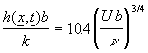For a 1000 rpm engine with a 0.1m stroke, combustion gas temperature of 1000C, and coolant temperature of 80 CWhere:

b = 0.1 m
k = 0.06 W/mK
n = 100 x 10 -6 m2/s

the average heat transfer coefficient is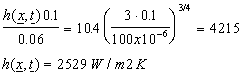The average heat transfer per unit area from the cylinder to the coolant is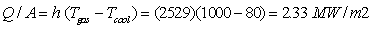For a reference cylinder area of p b2/4, the average heat transfer is